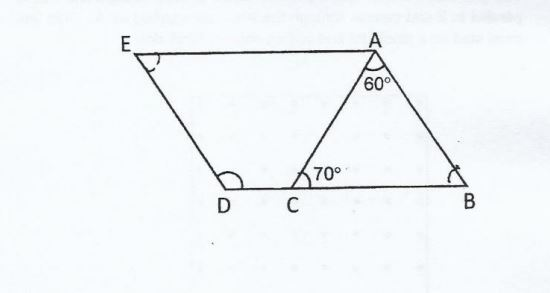# Question 1 of 100

In the figure below not, drawn to scale, ABDE is a parallelogram. $\angle$ ACB =70$^\circ$. and $\angle$ BAC = 60 $^\circ$. Find $\angle$ EDC.A
120$^\circ$
B
130$^\circ$
C
140$^\circ$
D
150$^\circ$
E
None of the above